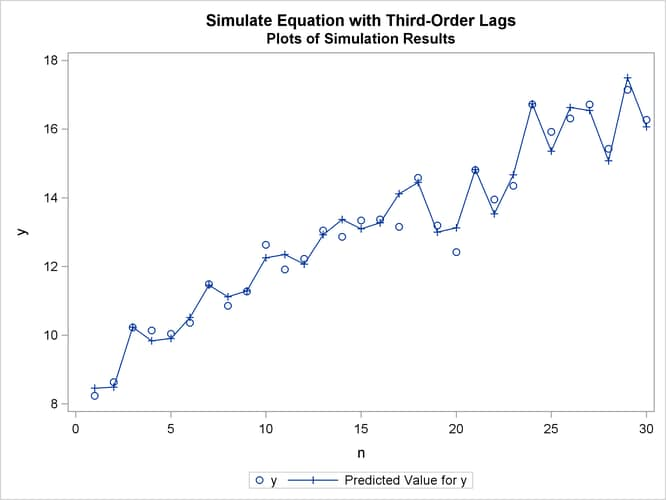# The SIMLIN Procedure

### Example 25.2 Multipliers for a Third-Order System

This example shows how to fit and simulate a single equation dynamic model with third-order lags. It then shows how to convert the third-order equation into a three equation system with only first-order lags, so that the SIMLIN procedure can compute multipliers. (See the section "Multipliers for Higher Order Lags" earlier in this chapter for more information.)

The input data set TEST is created from simulated data. A partial listing of the data set TEST produced by PROC PRINT is shown in Output 25.2.1.

Output 25.2.1: Partial Listing of Input Data Set

 Simulate Equation with Third-Order Lags Listing of Simulated Input Data

Obs y ylag1 ylag2 ylag3 x n
1 8.2369 8.5191 6.9491 7.8800 -1.2593 1
2 8.6285 8.2369 8.5191 6.9491 -1.6805 2
3 10.2223 8.6285 8.2369 8.5191 -1.9844 3
4 10.1372 10.2223 8.6285 8.2369 -1.7855 4
5 10.0360 10.1372 10.2223 8.6285 -1.8092 5
6 10.3560 10.0360 10.1372 10.2223 -1.3921 6
7 11.4835 10.3560 10.0360 10.1372 -2.0987 7
8 10.8508 11.4835 10.3560 10.0360 -1.8788 8
9 11.2684 10.8508 11.4835 10.3560 -1.7154 9
10 12.6310 11.2684 10.8508 11.4835 -1.8418 10

The REG procedure processes the input data and writes the parameter estimates to the OUTEST= data set A.

```title2 'Estimated Parameters';
proc reg data=test outest=a;
model y=ylag3 x;
run;

title2 'Listing of OUTEST= Data Set';
proc print data=a;
run;
```

Output 25.2.2 shows the printed output produced by the REG procedure, and Output 25.2.3 displays the OUTEST= data set A produced.

Output 25.2.2: Estimates and Fit Information from PROC REG

 Simulate Equation with Third-Order Lags Estimated Parameters

The REG Procedure
Model: MODEL1
Dependent Variable: y

Analysis of Variance
Source DF Sum of
Squares
Mean
Square
F Value Pr > F
Model 2 173.98377 86.99189 1691.98 <.0001
Error 27 1.38818 0.05141
Corrected Total 29 175.37196

 Root MSE R-Square 0.22675 0.9921 13.0523 0.9915 1.73721

Parameter Estimates
Variable DF Parameter
Estimate
Standard
Error
t Value Pr > |t|
Intercept 1 0.14239 0.23657 0.60 0.5523
ylag3 1 0.77121 0.01723 44.77 <.0001
x 1 -1.77668 0.10843 -16.39 <.0001

Output 25.2.3: The OUTEST= Data Set Created by PROC REG

 Simulate Equation with Third-Order Lags Listing of OUTEST= Data Set

Obs _MODEL_ _TYPE_ _DEPVAR_ _RMSE_ Intercept ylag3 x y
1 MODEL1 PARMS y 0.22675 0.14239 0.77121 -1.77668 -1

The SIMLIN procedure processes the TEST data set using the estimates from PROC REG. The following statements perform the simulation and write the results to the OUT= data set OUT2.

```title2 'Simulation of Equation';
proc simlin est=a data=test nored;
endogenous y;
exogenous  x;
lagged ylag3 y 3;
id n;
output out=out1 predicted=yhat residual=yresid;
run;
```

The printed output from the SIMLIN procedure is shown in Output 25.2.4.

Output 25.2.4: Output Produced by PROC SIMLIN

 Simulate Equation with Third-Order Lags Simulation of Equation

The SIMLIN Procedure

Fit Statistics
Variable N Mean Error Mean Pct
Error
Mean Abs Error Mean Abs
Pct Error
RMS
Error
RMS Pct
Error
y 30 -0.0233 -0.2268 0.2662 2.05684 0.3408 2.6159

The following statements plot the actual and predicted values, as shown in Output 25.2.5.

```title2 'Plots of Simulation Results';
proc sgplot data=out1;
scatter x=n y=y;
series x=n y=yhat / markers markerattrs=(symbol=plus);
run;
```

Output 25.2.5: Plot of Predicted and Actual ValuesNext, the input data set TEST is modified by creating two new variables, YLAG1X and YLAG2X, that are equal to YLAG1 and YLAG2. These variables are used in the SYSLIN procedure. (The estimates produced by PROC SYSLIN are the same as before and are not shown.) A listing of the OUTEST= data set B created by PROC SYSLIN is shown in Output 25.2.6.

```data test2;
set test;
ylag1x=ylag1;
ylag2x=ylag2;
run;
```
```title2 'Estimation of parameters and definition of identities';
proc syslin data=test2 outest=b;
endogenous y ylag1x ylag2x;
model y=ylag3 x;
identity ylag1x=ylag1;
identity ylag2x=ylag2;
run;

title2 'Listing of OUTEST= data set from PROC SYSLIN';
proc print data=b;
run;
```

Output 25.2.6: Listing of OUTEST= Data Set Created from PROC SYSLIN

 Simulate Equation with Third-Order Lags Listing of OUTEST= data set from PROC SYSLIN

Obs _TYPE_ _STATUS_ _MODEL_ _DEPVAR_ _SIGMA_ Intercept ylag3 x ylag1 ylag2 y ylag1x ylag2x
1 OLS 0 Converged y y 0.22675 0.14239 0.77121 -1.77668 . . -1 . .
2 IDENTITY 0 Converged   ylag1x . 0.00000 . . 1 . . -1 .
3 IDENTITY 0 Converged   ylag2x . 0.00000 . . . 1 . . -1

The SIMLIN procedure is used to compute the reduced form and multipliers. The OUTEST= data set B from PROC SYSLIN is used as the EST= data set for the SIMLIN procedure. The following statements perform the multiplier analysis.

```title2 'Simulation of transformed first-order equation system';

proc simlin est=b data=test2 total interim=2;
endogenous y ylag1x ylag2x;
exogenous  x;
lagged  ylag1 y 1  ylag2 ylag1x 1  ylag3 ylag2x 1;
id n;
output out=out2 predicted=yhat residual=yresid;
run;
```

Output 25.2.7 shows the interim 2 and total multipliers printed by the SIMLIN procedure.

Output 25.2.7: Interim 2 and Total Multipliers

 Simulate Equation with Third-Order Lags Simulation of transformed first-order equation system

The SIMLIN Procedure

Interim Multipliers for Interim 2
Variable x Intercept
y 0.000000 0.0000000
ylag1x 0.000000 0.0000000
ylag2x -1.776682 0.1423865

Total Multipliers
Variable x Intercept
y -7.765556 0.6223455
ylag1x -7.765556 0.6223455
ylag2x -7.765556 0.6223455